Enter parameters Horizontal Cylinder
Enter parameters
LENGTH, WIDTH & DEPTH
Diameter:
in
• inches (in)
• feet (ft)
• yards (yd)
• centimeters (cm)
• meters (m)
Length:
in
• inches (in)
• feet (ft)
• yards (yd)
• centimeters (cm)
• meters (m)
Filled Depth:
in
• inches (in)
• feet (ft)
• yards (yd)
• centimeters (cm)
• meters (m)
Reset
Result
Liquid Capacity:
0
US gal
• US Gallons (US gal)
• UK Gallons (UK gal)
• Barrels (bbl)
• Liters (l)
Tank volume:
0
ft³
• cubic yards (yd³)
• cubic feet (ft³)
• cubic inches (in³)
• cubic centimeters (cm³)
• cubic meters ()
Filled Volume:
0
US gal
• US Gallons (US gal)
• UK Gallons (UK gal)
• Barrels (bbl)
• Liters (l)
• cubic inches (in³)
• cubic feet (ft³)
• cubic meters ()
Enter parameters
LENGTH, WIDTH & DEPTH
Diameter:
in
• inches (in)
• feet (ft)
• yards (yd)
• centimeters (cm)
• meters (m)
Height:
in
• inches (in)
• feet (ft)
• yards (yd)
• centimeters (cm)
• meters (m)
Filled Depth:
in
• inches (in)
• feet (ft)
• yards (yd)
• centimeters (cm)
• meters (m)
Reset
Result
Liquid Capacity:
0
US gal
• US Gallons (US gal)
• UK Gallons (UK gal)
• Barrels (bbl)
• Liters (l)
Tank volume:
0
ft³
• cubic yards (yd³)
• cubic feet (ft³)
• cubic inches (in³)
• cubic centimeters (cm³)
• cubic meters ()
Filled Volume:
0
US gal
• US Gallons (US gal)
• UK Gallons (UK gal)
• Barrels (bbl)
• Liters (l)
• cubic inches (in³)
• cubic feet (ft³)
• cubic meters ()
Enter parameters
LENGTH, WIDTH & DEPTH
Width:
in
• inches (in)
• feet (ft)
• yards (yd)
• centimeters (cm)
• meters (m)
Height:
in
• inches (in)
• feet (ft)
• yards (yd)
• centimeters (cm)
• meters (m)
Length:
in
• inches (in)
• feet (ft)
• yards (yd)
• centimeters (cm)
• meters (m)
Filled Depth:
in
• inches (in)
• feet (ft)
• yards (yd)
• centimeters (cm)
• meters (m)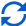Result
Liquid Capacity:
0
US gal
• US Gallons (US gal)
• UK Gallons (UK gal)
• Barrels (bbl)
• Liters (l)
Tank volume:
0
ft³
• cubic yards (yd³)
• cubic feet (ft³)
• cubic inches (in³)
• cubic centimeters (cm³)
• cubic meters ()
Filled Volume:
0
US gal
• US Gallons (US gal)
• UK Gallons (UK gal)
• Barrels (bbl)
• Liters (l)
• cubic inches (in³)
• cubic feet (ft³)
• cubic meters ()
Enter parameters
LENGTH, WIDTH & DEPTH
Diameter:
in
• inches (in)
• feet (ft)
• yards (yd)
• centimeters (cm)
• meters (m)
Side Length:
in
• inches (in)
• feet (ft)
• yards (yd)
• centimeters (cm)
• meters (m)
Filled Depth:
in
• inches (in)
• feet (ft)
• yards (yd)
• centimeters (cm)
• meters (m)Result
Liquid Capacity:
0
US gal
• US Gallons (US gal)
• UK Gallons (UK gal)
• Barrels (bbl)
• Liters (l)
Tank volume:
0
ft³
• cubic yards (yd³)
• cubic feet (ft³)
• cubic inches (in³)
• cubic centimeters (cm³)
• cubic meters ()
Filled Volume:
0
US gal
• US Gallons (US gal)
• UK Gallons (UK gal)
• Barrels (bbl)
• Liters (l)
• cubic inches (in³)
• cubic feet (ft³)
• cubic meters ()
Enter parameters
LENGTH, WIDTH & DEPTH
Diameter:
in
• inches (in)
• feet (ft)
• yards (yd)
• centimeters (cm)
• meters (m)
Side Height:
in
• inches (in)
• feet (ft)
• yards (yd)
• centimeters (cm)
• meters (m)
Filled Depth:
in
• inches (in)
• feet (ft)
• yards (yd)
• centimeters (cm)
• meters (m)Result
Liquid Capacity:
0
US gal
• US Gallons (US gal)
• UK Gallons (UK gal)
• Barrels (bbl)
• Liters (l)
Tank volume:
0
ft³
• cubic yards (yd³)
• cubic feet (ft³)
• cubic inches (in³)
• cubic centimeters (cm³)
• cubic meters ()
Filled Volume:
0
US gal
• US Gallons (US gal)
• UK Gallons (UK gal)
• Barrels (bbl)
• Liters (l)
• cubic inches (in³)
• cubic feet (ft³)
• cubic meters ()
Enter parameters
LENGTH, WIDTH & DEPTH
Width:
in
• inches (in)
• feet (ft)
• yards (yd)
• centimeters (cm)
• meters (m)
Height:
in
• inches (in)
• feet (ft)
• yards (yd)
• centimeters (cm)
• meters (m)
Length:
in
• inches (in)
• feet (ft)
• yards (yd)
• centimeters (cm)
• meters (m)
Filled Depth:
in
• inches (in)
• feet (ft)
• yards (yd)
• centimeters (cm)
• meters (m)Result
Liquid Capacity:
0
US gal
• US Gallons (US gal)
• UK Gallons (UK gal)
• Barrels (bbl)
• Liters (l)
Tank volume:
0
ft³
• cubic yards (yd³)
• cubic feet (ft³)
• cubic inches (in³)
• cubic centimeters (cm³)
• cubic meters ()
Filled Volume:
0
US gal
• US Gallons (US gal)
• UK Gallons (UK gal)
• Barrels (bbl)
• Liters (l)
• cubic inches (in³)
• cubic feet (ft³)
• cubic meters ()
Enter parameters
LENGTH, WIDTH & DEPTH
Width:
in
• inches (in)
• feet (ft)
• yards (yd)
• centimeters (cm)
• meters (m)
Length:
in
• inches (in)
• feet (ft)
• yards (yd)
• centimeters (cm)
• meters (m)
Height:
in
• inches (in)
• feet (ft)
• yards (yd)
• centimeters (cm)
• meters (m)
Filled Depth:
in
• inches (in)
• feet (ft)
• yards (yd)
• centimeters (cm)
• meters (m)Result
Liquid Capacity:
0
US gal
• US Gallons (US gal)
• UK Gallons (UK gal)
• Barrels (bbl)
• Liters (l)
Tank volume:
0
ft³
• cubic yards (yd³)
• cubic feet (ft³)
• cubic inches (in³)
• cubic centimeters (cm³)
• cubic meters ()
Filled Volume:
0
US gal
• US Gallons (US gal)
• UK Gallons (UK gal)
• Barrels (bbl)
• Liters (l)
• cubic inches (in³)
• cubic feet (ft³)
• cubic meters ()
Enter parameters
Top Diameter:
in
• inches (in)
• feet (ft)
• yards (yd)
• centimeters (cm)
• meters (m)
Bottom Diameter:
in
• inches (in)
• feet (ft)
• yards (yd)
• centimeters (cm)
• meters (m)
Cylinder Height:
in
• inches (in)
• feet (ft)
• yards (yd)
• centimeters (cm)
• meters (m)
Frustum Height:
in
• inches (in)
• feet (ft)
• yards (yd)
• centimeters (cm)
• meters (m)
Filled Depth:
in
• inches (in)
• feet (ft)
• yards (yd)
• centimeters (cm)
• meters (m)Result
Liquid Capacity:
0
US gal
• US Gallons (US gal)
• UK Gallons (UK gal)
• Barrels (bbl)
• Liters (l)
Tank volume:
0
ft³
• cubic yards (yd³)
• cubic feet (ft³)
• cubic inches (in³)
• cubic centimeters (cm³)
• cubic meters ()
Filled Volume:
0
US gal
• US Gallons (US gal)
• UK Gallons (UK gal)
• Barrels (bbl)
• Liters (l)
• cubic inches (in³)
• cubic feet (ft³)
• cubic meters ()
Embed(6 votes, average: 5.00 out of 5)Loading...
Similar Calculators:
Real-time graphics. Make the calculations and see the changes.

Got it
Real-time graphics. Make the calculations and see the changes.

Got it
Real-time graphics. Make the calculations and see the changes.

Got it
Real-time graphics. Make the calculations and see the changes.

Got it
Real-time graphics. Make the calculations and see the changes.

Got it
Real-time graphics. Make the calculations and see the changes.

Got it
Real-time graphics. Make the calculations and see the changes.

Got it
Real-time graphics. Make the calculations and see the changes.

Got it
Embed
Embed
Similar Calculators:We understand that it isn’t very easy to estimate how many gallons or litres your container can hold.

That’s why we built our online tank volume calculator. 🤘

Keep reading to see how using our capacity calculator makes calculating the volume of your tank easy.

But first…

Contents:

## Tank volume calculator formula

Calculate the volume of liquid your container can hold by entering your dimensions in metric units (centimeters or meters) or imperial units (yards, feet or inches).

Our tool estimates the total tank volume and liquid capacity using the below formulas:

### Horizontal Cylindrical TankThe total volume of a horizontal cylindrical tank is calculated by using the formula:

$$Volume = \pi × Radius^2 × Length$$

Where

$$Radius = {Diameter \over 2}$$

###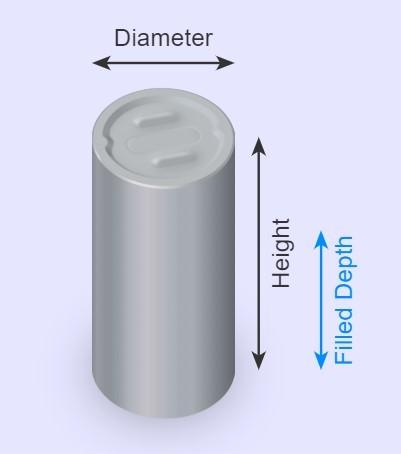The total volume of a vertical cylindrical tank is calculated by using the formula:

$$Volume = \pi × Radius^2 × Height$$

Where

$$Radius = {Diameter \over 2}$$

### Rectangular TankThe total volume of a rectangular tank is calculated by using the formula:

$$Volume = Length × Width × Height$$

###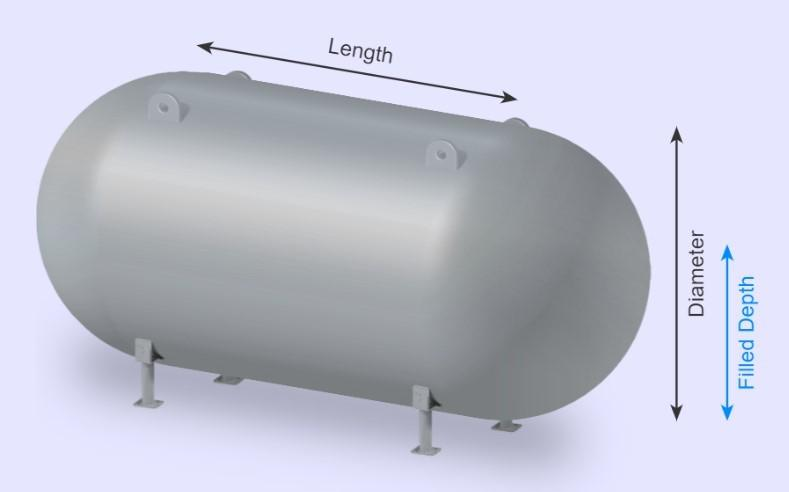The total volume of a capsule tank is calculated by using the formula:

$$Volume= \pi × Radius^2 × \biggl(Side\,Length+ {4 × Radius \over 3}\biggr)$$

Where

$$Radius = {Diameter \over 2}$$

### Vertical Capsule TankThe total volume of a capsule tank is calculated by using the formula:

$$Volume= \pi × Radius^2 × \biggl(Side\,Height+ {4 × Radius \over 3}\biggr)$$

Where

$$Radius = {Diameter \over 2}$$

###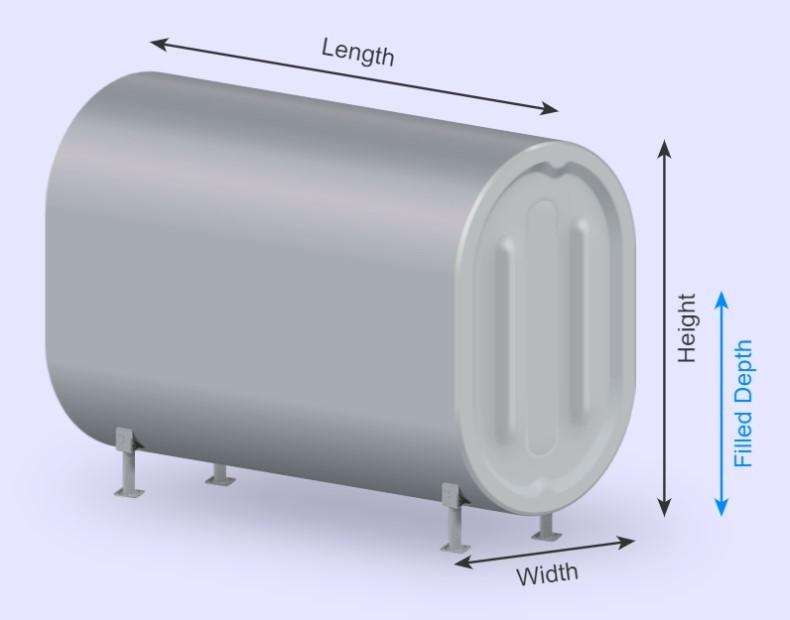The total volume of an oval tank is calculated by using the formula:

$$Volume = Length × (π × Radius^2 + Width × Y)$$

Where

$$Radius = {Width \over 2}$$

and

$$Y = Height – Width \quad if\,Height > Width$$

$$Y = Width – Height \quad if\,Width > Height$$

### Vertical Oval TankThe total volume of an oval tank is calculated by using the formula:

$$Volume = Height × (π × Radius^2 + Width × Y)$$

Where

$$Radius = {Width \over 2}$$

and

$$Y = Length – Width \quad if\,Length > Width$$

$$Y = Width – Length \quad if\,Width > Length$$

###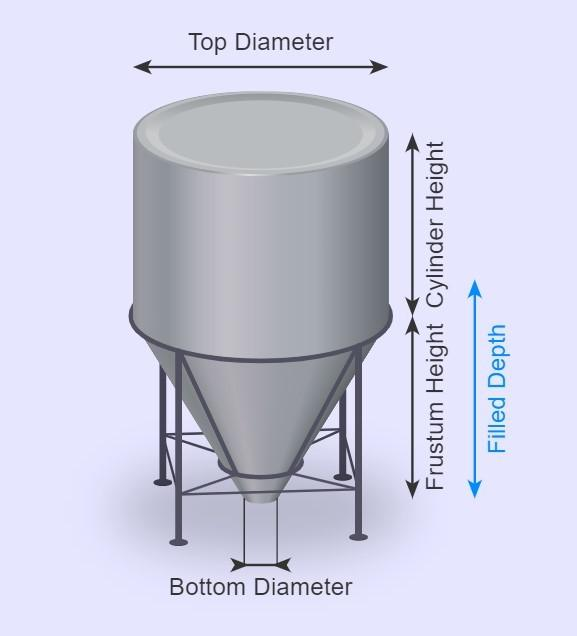The total volume of a cone bottom tank is calculated by using the formula:

$$Volume = Volume\,Cylinder + Volume\,Frustrum$$

Where

$$Volume\,Cylinder = \pi × Top\,Radius^2 × Cylinder\,Height$$

And

$$Volume\,Frustrum = {1 \over 3} × \pi × Frustrum\,Height × (Top\,Radius^2 + Bottom\,Radius^2 + Top\,Radius × Bottom\,Radius)$$

With

$$Top\,Radius = {Top\,Diameter \over 2}$$

and

$$Bottom\,Radius = {Bottom\,Diameter \over 2}$$

## How to find the volume of liquid in partially filled tank

Our tank volume calculator also has an option for a tank that is only partially filled.

The formulae start to become more complicated when we look at tanks that are only partially filled, so make sure you check the values carefully – using our calculator can help you simplify the process! 😉

Below are the formulae the calculator uses to work out the volume of water or fuel in a partially filled tank:

### Partially Filled Horizontal Circular Tank

The volume of water, filled to a certain depth, is given by:

$$Filled\,Volume = {1 \over2} × Radius^2 × (x – sin\,⁡x ) × Length$$

Where

$$x = 2 × cos^{-1} \biggl({Radius – Filled\,Depth \over Radius}\biggr)$$

and

$$Radius = {Diameter \over 2}$$

### Partially Filled Vertical Circular Tank

$$Filled\,Volume = \pi × Radius^2 × Filled\,Depth$$

Where

$$Radius = {Diameter \over 2}$$

### Partially Filled Rectangular Tank

$$Filled\,Volume = Length × Width × Filled\,Depth$$

### Partially Filled Horizontal Oval Tank

$$Filled\,Volume = {1 \over 2} × Radius^2 × (x – sin⁡\,x) × Length + (Length × Filled\,Depth × (Width – Height))$$

$$x = 2 × cos^{-1} \biggl({Radius – Filled\,Depth \over Radius}\biggr)$$

and

$$Radius = {Width \over 2}$$

### Partially Filled Vertical Oval Tank

• If Filled Depth < Radius then:

$$Filled\,Volume = {1 \over 2} × Radius^2 × (x – sin⁡\,x) × Length$$

Where

$$x = 2 × cos^{-1} \biggl({Radius – Filled\,Depth \over Radius}\biggr)$$

and

$$Radius = {Width \over 2}$$

• If Radius < Filled Depth < (Height – Width) then:

$$Filled\,Volume = {1 \over 2} × \pi × Radius^2 × Length + (Filled\,Depth – Radius) × Length × Width$$

Where

$$Radius = {Width \over 2}$$

• If (Height – Radius) < Filled Depth < Height then:

$$Filled\,Volume = Total\,Liquid\,Capacity – Empty\,Portion$$

Where

$$Empty\,Portion = \pi × Radius^2 × Length – {1 \over 2} × Radius^2 × (x – sin\,x) × Length$$

and

$$x = 2 × cos^{-1} \biggl({Radius – Filled\,Depth \over Radius}\biggr)$$

and

$$Radius = {Width \over 2}$$

### Partially Filled Horizontal Capsule

$$Filled\,Volume = Filled\,Cylinder + Filled\,Spherical\,Ends$$

Therefore

$$Filled\,Volume = {1 \over 2} × Radius^2 × (x – sin\,x) × Length + {\pi × Filled\,Depth^2 \over 3} × (1.5 × Diameter – Filled\,Depth)$$

Where

$$x = 2 × cos^{-1} \biggl({Radius – Filled\,Depth \over Radius}\biggr)$$

and

$$Radius = {Diameter \over 2}$$

### Partially Filled Vertical Capsule

• If the tank is filled to below the start of the cylindrical part of the tank i.e.

$$Filled\,Depth < {Diameter \over 2}$$

then:

$$Filled\,Volume = {\pi × Filled\,Depth^2 \over 3} × (1.5 × Diameter – Filled\,Depth)$$

• If the tank is filled to under the end of the cylindrical part of the tank, i.e.

$${Diameter \over 2} < Filled\,Depth < {Diameter \over 2} + Length$$

then:

$$Filled\,Volume = {2 \over 3} × \pi × Radius^3 + \pi × Radius^2 × {Filled\,Depth – Diameter \over 2}$$

• If the tank is filled to above the end of the cylindrical part of the tank, i.e.

$${Diameter \over 2} + Length < Filled\,Depth$$

then:

$$Filled\,Volume = Total\,Capsule\,Volume – Spherical\,Cap$$

So

$$Filled\,Volume = Capsule\,Volume – {1 \over 3} × \pi × Filled\,Depth^2 × (1.5 × Diameter – (Length + Diameter – Filled\,Depth))$$

### Partially Filled Cone Bottom

• If the tank is filled to below the start of the cylinder, i.e.

$$Filled\,Depth < Frustum\,Height$$

Then, the total volume of the water contained in the tank is given by calculating the volume of the frustum filled:

$$Filled\,Volume = {1 \over 3} × \pi × Frustum\,Height × (R^2 + R × Bottom\,Radius + Bottom\,Radius^2 )$$

Where

$$Bottom\,Radius = {Bottom\,Diameter \over 2}$$

and

$$R = {1 \over 2} × Top\,Diameter × {Filled\,Depth + Z \over Frustum\,Height+Z}$$

Where

$$Z = Missing\,Cone\,Height$$

• If the tank is filled to above the start of the cylinder, i.e.

$$Frustum\,Height < Filled\,Depth$$

Then

$$Filled\,Volume = Cylindrical\,Filled\,Volume + Frustrum\,Volum$$

Where

$$Cylindrical\,Filled\,Volume = \pi × Radius^2 × (Filled\,Depth – Frustum\,Height)$$

Confused? 🤔

See below for four full examples showing in detail how our calculator works.

Otherwise, simply enter your measurements for your container in our tank size calculator!

## Example calculations

✅ Cylindrical Oil Tank

• Let’s say that I have a cylindrical oil tank which measures 7 yards in length and has a round face 5 feet in diameter (the distance across the circular end passing through the central point).
• I want to calculate the tank volume in cubic feet and work out how much oil will fit in the cylinder in US gallons.
• I would enter the values as shown in the calculator and select the correct units in the drop down options for measurements.
• The cylindrical tank calculator would then perform the following operations to calculate the size of the cylindrical oil tank:

$$Volume = \pi × Radius^2 × Length$$

Where

$$Radius = {Diameter \over 2}$$

Therefore

$$Volume = \pi × {5 ft \over 2}^2 × 7\,yd = 412.3\,ft^3 = 3084\,US\,Gal$$

Now, let’s say we filled the cylindrical tank to a height of 2 feet. The total water in the tank is calculated by:

$$Filled\,Volume = {1 \over2} × Radius^2 × (x – sin\,⁡x ) × Length$$

Where

$$x = 2 × cos^{-1} \biggl({Radius – Filled\,Depth \over Radius}\biggr) = 2 × cos^{-1} \biggl({2.5\,ft – 2\,ft \over 2.5\,ft}\biggr) = 156.9$$

and

$$Radius = {Diameter \over 2} = {5\,ft \over 2} = 2.5\,ft$$

Therefore

$$Filled\,Volume = {1 \over2} × 2.5\,ft^2 × (156.9 – sin\,⁡156.9 ) × 7\,yd = 154\,ft^3 = 1152.1\,US\,Gal$$

Rectangular Fuel Tank

• Let’s say that I have a rectangular fuel tank which measures 4 feet wide, 2 feet in length and has a vertical height of 10 inches.
• I want to calculate the tank volume in cubic feet and work out how much fuel will fit in my tank in barrels (bbl).
• I would enter the values in the fields and select the correct units in the drop down options for measurement.
• The calculator would then perform the following calculations:

$$Volume = Length × Width × Height = 2\,ft × 4\,ft × 10\,in = 6.667\,ft^3 = 1.187\,bbl$$

Now, let’s say that we filled the fuel tank to a height of 3 inches.

To calculate the total volume of the liquid the calculator would do the following operations:

$$Filled\,Volume = Length × Width × Filled\,Depth = 2\,ft × 4\,ft × 3\,in = 2\,ft^3 = 14.96\,US\,Gal$$

✅ Horizontal Capsule Tank

• Let’s now say that I have a water capsule tank (a cylindrical tank with circular ends) which measures 10 inches in diameter and 30 inches in horizontal side length.
• I want to know its volume in cubic inches and therefore its liquid capacity (how much water I can fit in the tank) in litres.
• I would enter the values in the calculator as shown in the figure above, selecting the correct units for each measurement from the drop down options.
• Then it would perform the following calculations to work out the volume of the tank:

$$Volume= \pi × Radius^2 × \biggl(Length+ {4 × Radius \over 3}\biggr)$$

Where

$$Radius = {Diameter \over 2}$$

Therefore

$$Volume= \pi × \biggl({10\,in \over 2}\biggr)^2 × \biggl(30\,in + {4 \over 3} × {10\,in \over 2}\biggr) = \pi × 5\,in^2 × \biggl(30\,in + {20\,in \over 3}\biggr) = 2879.793\,in^3 = 47.2\,l$$

Therefore, my tank measures approximately 2880 cubic inches and I can fill it with 47.2 liters of water.

Now, let’s say that I want to fill the tank to a depth of 3 inches.

To calculate the total amount of liquid in the tank, the calculator would do the following calculations:

$$Filled\,Volume = {1 \over 2} × Radius^2 × (x – sin\,x) × Length + {\pi × Filled\,Depth^2 \over 3} × (1.5 × Diameter – Filled\,Depth)$$

Where

$$x = 2 × cos^{-1} \biggl({Radius – Filled\,Depth \over Radius}\biggr) = 2 × cos^{-1} \biggl({5\,in – 3\,in \over 5\,in}\biggr) = 132.84$$

and

$$Radius = {10\,in \over 2} = 5\,in$$

Therefore

$$Filled\,Volume = {1 \over 2} × 5\,in^2 × (132.84 – sin\,132.84) × 30\,in + {\pi × 3\,in^2 \over 3} × (1.5 × 10\,in – 3\,in) = 0.409\,ft^3 = 3.06\,US\,Gal$$

✅ Horizontal Oval Tank

• For the final example, let’s imagine that I have an oval tank which is 7 feet long horizontally.
• The oval face measures 100 cm wide and has a vertical height of 4 feet.
• I want to know how many litres of water I can fill my tank with and what the tank volume is in cubic yards.
• I would enter the correct values in the calculator, selecting the appropriate units for each measurement from the drop down options.
• It would perform the following calculations:

$$Volume = Length × (π × Radius^2 + Width × Y)$$

Where

$$Radius = {Width \over 2}$$

and

$$Y = Height – Width \quad if\,Height > Width$$

$$Y = Width – Height \quad if\,Width > Height$$

Therefore

$$Volume = 7\,ft × (π × 50\,cm^2 + 100\,cm × (4\,ft – 100\,cm)) = 2.803\,yd^3 = 2143.411\,litres$$

Note that we use Y = Height – Width because height (4 ft = 121cm) is larger than 100 cm.

Now, let’s say I have filled the tank with water to a depth of 1.5 ft.

The calculator works out the total volume of water by doing  the following calculations:

$$Filled\,Volume = {1 \over 2} × Radius^2 × (x – sin⁡\,x) × Length + (Length × Filled\,Depth × (Width – Height))$$

Where

$$x = 2 × cos^{-1} \biggl({Radius – Filled\,Depth \over Radius}\biggr) = 2 × cos^{-1} \biggl({50\,cm – 1.5\,ft \over 50\,cm}\biggr) = 170.2$$

and

$$Radius = {Width \over 2} = {100\,cm \over 2} = 50\,cm$$

Therefore

$$Filled\,Volume = {1 \over 2} × 50\,cm^2 × (170.2 – sin⁡\,170.2) × 7\,ft + (7\,ft × 1.5\,ft × (100\,cm – 4\,ft)) = 26.37\,ft^3 = 197.25\,US\,Gallons$$

At this point, it’s likely that you are asking the following question:

## What if my dimensions are in different units?

The best part of our online calculator is that it takes care of this for you! 😉

Looking through our examples, perhaps you noticed that we changed units between feet, centimeters, inches and so on, e.g. in example 2:

$$Volume = Length × Width × Height = 2\,ft × 4\,ft × 10\,in = 6.667\,ft^3 = 1.187\,bbl$$

We can do this because our calculator is able to do the conversions for you, making it far easier for you!

For each measurement there are multiple options that are available to use. For example, length can be calculated in terms of feet (ft), inches (in), yards (yd), meters (m) or centimeters (cm).

The calculator takes care of this by using the following conversions:

$$1\,foot = 12\,inches = 0.33\,yards = 30.48\,centimeters = 0.3048\,meters$$

$$1\,ft^3 = 1728\,in^3 = 0.037\,yd^3 = 28316.8\,cm^3 = 0.0283168\,m^3$$

Note that the default value for all lengths is inches, tank volume is cubic inches and liquid capacity is US gallons.

Each of these can be changed by pressing the arrows next to the unit measurements and selecting the correct unit from the drop down options.

We think that our tank volume calculator is an effective and powerful online tool. It makes calculating the volume of your tank very easy! 😊

Embed this calculator on your site!Add live graphics
Copied to clipboard! Preview